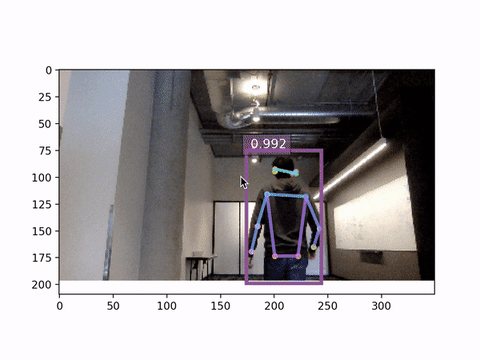# 3. Estimate pose from your webcam¶

First, import the necessary modules.

from __future__ import division
import argparse, time, logging, os, math, tqdm, cv2

import numpy as np
import mxnet as mx
from mxnet import gluon, nd, image
from mxnet.gluon.data.vision import transforms

import matplotlib.pyplot as plt

import gluoncv as gcv
from gluoncv import data
from gluoncv.data import mscoco
from gluoncv.model_zoo import get_model
from gluoncv.data.transforms.pose import detector_to_simple_pose, heatmap_to_coord
from gluoncv.utils.viz import cv_plot_image, cv_plot_keypoints


In this tutorial we feed frames from the webcam into a detector, then we estimate the pose for each detected people in the frame.

For the detector we use ssd_512_mobilenet1.0_coco as it is fast and accurate enough.

ctx = mx.cpu()
detector_name = "ssd_512_mobilenet1.0_coco"
detector = get_model(detector_name, pretrained=True, ctx=ctx)


The pre-trained model tries to detect all 80 classes of objects in an image, however in pose estimation we are only interested in one object class: person.

To speed up the detector, we can reset the prediction head to only include the classes we need.

detector.reset_class(classes=['person'], reuse_weights={'person':'person'})
detector.hybridize()


Next for the estimator, we choose simple_pose_resnet18_v1b for it is light-weighted.

The default simple_pose_resnet18_v1b model was trained with input size 256x192. We also provide an optional simple_pose_resnet18_v1b model trained with input size 128x96. The latter one is going to be faster, which means a smoother webcam demo. Remember that we can load an optional pre-trained model by passing its shasum to pretrained.

estimator = get_model('simple_pose_resnet18_v1b', pretrained='ccd24037', ctx=ctx)
estimator.hybridize()


With OpenCV, we can easily retrieve frames from the webcam.

cap = cv2.VideoCapture(0)
time.sleep(1)  ### letting the camera autofocus


Note

In the code we run the demo on CPU, if your machine has a GPU then you may try heavier and more accurate pre-trained detectors and estimators.

For a list of models to choose from, please refer to our Model Zoo pages for detection and pose estimation.

## Estimation loop¶

For each frame, we perform the following steps:

• pre-process the image

• detect people in the image

• post-process the detected people

• estimate the pose for each person

• plot the result

axes = None
num_frames = 100

for i in range(num_frames):
frame = mx.nd.array(cv2.cvtColor(frame, cv2.COLOR_BGR2RGB)).astype('uint8')

x, frame = gcv.data.transforms.presets.ssd.transform_test(frame, short=512, max_size=350)
x = x.as_in_context(ctx)
class_IDs, scores, bounding_boxs = detector(x)

pose_input, upscale_bbox = detector_to_simple_pose(frame, class_IDs, scores, bounding_boxs,
output_shape=(128, 96), ctx=ctx)
if len(upscale_bbox) > 0:
predicted_heatmap = estimator(pose_input)
pred_coords, confidence = heatmap_to_coord(predicted_heatmap, upscale_bbox)

img = cv_plot_keypoints(frame, pred_coords, confidence, class_IDs, bounding_boxs, scores,
box_thresh=0.5, keypoint_thresh=0.2)
cv_plot_image(img)
cv2.waitKey(1)


We release the webcam before exiting:

cap.release()


## Results¶

Download cam_demo.py

Run the script

python cam_demo.py --num-frames 100


If all goes well you should be able to see your pose detected!The input size significantly affect the inference speed. Below is the webcam demo with input 256x192, compare the frames per second!Total running time of the script: ( 0 minutes 0.000 seconds)

Gallery generated by Sphinx-Gallery